1. A student states that the following factors may lead to global warming

I. decreased albedo of the Earth’s surface
II. increase in volcanic activity
III. deforestation.

Which of the above statements are correct?

2. The surface temperature of a black-body emitter is doubled. By what factor does the power emitted by the body increase?
3. The greenhouse effect can be explained by the fact that the infrared radiation emitted by the surface of Earth
4. The original source of the electrical power produced by a wind generator is
5. What is the correct order of energy transformations in a coal power station?
6. Which of the following processes leads to the production of a nucleus of plutonium-239 from a nucleus of uranium-238?
7. Large areas of rainforests are cut down and burned every year. The result of these actions is
8. In a nuclear fission reaction neutrons are passed through a moderator. The reason for this is to reduce the
9. Kindly refer the question below:

A spherical black body has absolute temperature T1. The surroundings are kept at a lower absolute temperature T2. What is the net power per unit area lost by the body?

10. A room is at a constant temperature of 300 K. A hotplate in the room is at a temperature of 400 K and loses energy by radiation at a rate of P. What is the rate of loss of energy from the hotplate when its temperature is 500 K?
11. The diagram shows an energy balance climate model for a planet.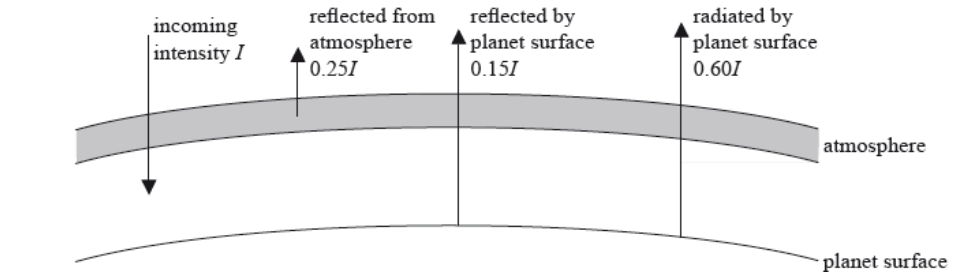The intensities of the reflected and radiated radiation are given in terms of the incident intensity . Which of the following is the albedo of this planet?

12. Which of the following is a renewable and non-renewable energy source?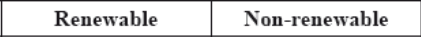13. In which of the following places will the albedo be greatest?
14. Which of the following is most likely to reduce the enhanced greenhouse effect?
15. The albedo for the oceans is lower than that for glaciers. This is because, compared to ice, sea water
16. Which type of power-production system is most suitable for responding to a sudden high increase in demand for electrical power?
17. Kindly refer the question below:

A solar panel has surface area 0.40m2 and efficiency 50%. The average intensity of radiation reaching the surface of the panel is 0.25kWm–2. What is the average power output from an array of 10 of these solar panels?

18. Which of the following energy sources results from the solar energy incident on Earth?
19. A wind turbine produces a power P when the wind speed is v. Assuming that the efficiency of the turbine is constant, the best estimate for the power produced when the wind speed becomes 2v is
20. Which of the following is the primary function of the moderator in a nuclear power station?
21. Wind of speed v is incident normally on a wind turbine of radius r.

The maximum theoretical power output of the turbine is P. For wind of speed 2v incident normally on a similar turbine of radius 1/2r, the maximum theoretical power will be

22. A black body has absolute temperature T and surface area A. The intensity of the radiation emitted by the body is I. Another black body of surface area 2A has absolute temperature 2T. What is the intensity of radiation emitted by this second black body?
23. The main role of a moderator in a nuclear fission reactor is to
24. Which of the energy sources are classified as renewable and non-renewable?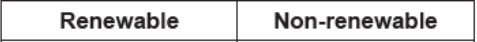25. Planet X and planet Y both emit radiation as black bodies. Planet X has a surface temperature that is less than the surface temperature of planet Y.

What is the graph of the variation of intensity I with wavelength λ for the radiation emitted by planet Y? The graph for planet X is shown dotted.

26. Increasing the temperature of a black-body will have the following effect on its emission spectrum.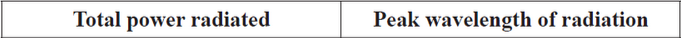27. Kindly refer the question below:

A black body of surface 1.0m2 emits electromagnetic radiation of peak wavelength 2.90×10–6m. Which of the following statements about the body are correct?

I.    The temperature of the body is 1000 K.
II.   The energy radiated by the body in one second is 5.7×104 J.
III.  The body is a perfect absorber of electromagnetic radiation.

28. The following are energy sources.

I.    a battery of rechargeable electric cells
II.   crude oil
III.  a pumped storage hydroelectric system

Which of these are secondary energy sources?

29. Which of the following is not a primary energy source?
30. The blades of a certain wind turbine X have radius r. The maximum theoretical available wind power for a given wind speed is P. Another similar turbine Y has blades of radius 2r. What is the best estimate for the maximum theoretical available wind power from turbine Y?
31. Which of the following correctly describes the energy transformation within photovoltaic cells and within solar heating panels?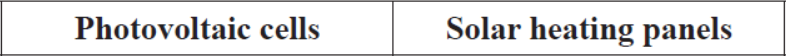32. What are the principal energy changes in a photovoltaic cell and in a solar heating panel?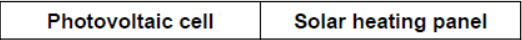33. Two pulses are travelling towards each other.What is a possible pulse shape when the pulses overlap?

34. The Sankey diagram represents the energy flow for a coal-fired power station.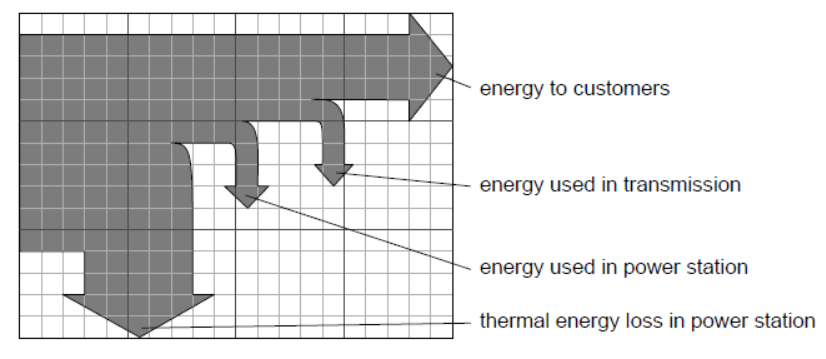What is the overall efficiency of the power station?

35. The Earth rotates about an axis XY, as shown below.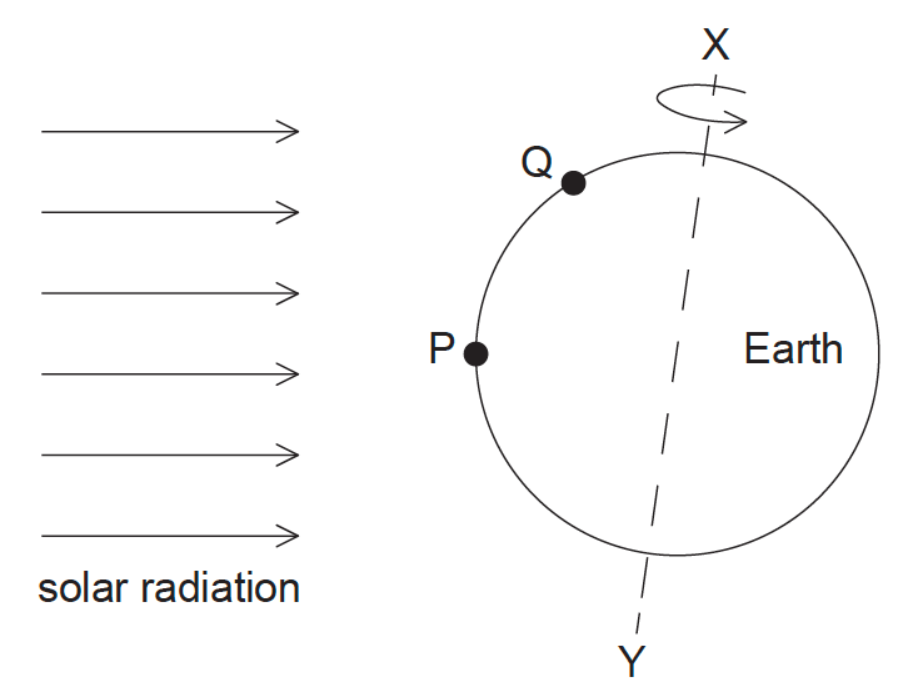P and Q are positions on the Earth’s surface that receive solar radiation from the Sun. Why is the intensity of the solar radiation incident at P significantly greater than the intensity at Q?

36. The graph shows the spectrum of a black-body.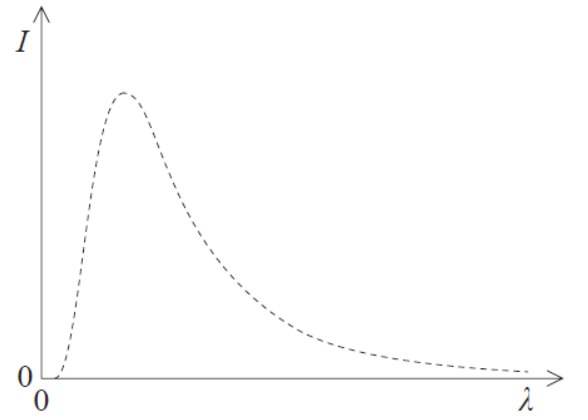Which graph shows the spectrum of a body of emissivity 0.5 at the same temperature as the black-body? (The original graph is shown dotted.)

37. What is the main role of the control rods and the main role of the moderator in a thermal fission reactor?